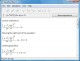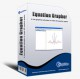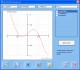Equation Wizard 1.21 Automatically solves algebraic equations of any order written in any form SharewarePDF Equation 0.7 PDF Equation gives you quick access to LaTeX's typesetting power in almost every Mac OS X application. FreewareEquation Service 1.0 Equation Service is a program that uses pdflatex to produce small PDF files containing equations and other text. FreewareChemical Equation Expert 2.12a Chemical Equation Expert is an integrated tool for chemistry professionals FreewareRapid-Pi 2.1 MS Word add-on that provides a faster way of editing mathematical equations SharewareMathMagic Pro for Quark 5.51 MathMagic Pro Edition is an equation editor mainly for use with QuarkXPress software in editing any mathematical expressions and symbols with WYSIWYG interface and various powerful features. DemoEquation Grapher 2.2 Equation grapher is a free graphing calculator to graph equations. FreewareDeadLine 2.36 Analyze, solve, and plot various mathematical equations. FreewareQuotient 1.4 Have you ever wondered what happens to the quotient of a division equation that goes off the screen of your calculator, such as 1/7? How long is it? Does it repeat? It does indeed repeat. FreewareMathMagic Pro for QuarkXPress 5.41 MathMagic Pro Edition for QuarkXPress is an equation editor mainly for use with QuarkXPress v5. Shareware